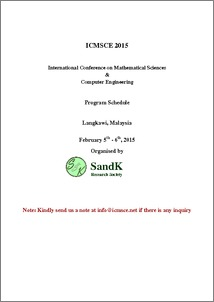# Cubic equations associated with p-adic potts models

Saburov, Mansoor (2015) Cubic equations associated with p-adic potts models. In: The 2nd International Conference on Mathematical Sciences and Computer Engineering, 5-6 Feb 2015, Langkawi, Kedah Darul Aman, Malaysia.Preview
PDFPDF (Program book, certificate & presentation) - Published Version
Restricted to Repository staff only

## Abstract

The p-adic models of statistical mechanics require the investigation of roots of polynomial equations over p-adic fields in order to construct p-adic Gibbs measures. The most frequently asked question is that whether a root of a polynomial equation belongs to some given domains. In this paper, we are aiming to study the solvability of general cubic equations over the set Z_p^{*} wherep>3. Our investigations enable to describe all translation invariant p-adic Gibbs measures on a Cayley tree of order three.

Item Type: Conference or Workshop Item (Plenary Papers) 6769/46249 Cubic equations, p-adic potts models Q Science > QA Mathematics Kulliyyah of Science > Department of Computational and Theoretical Sciences Dr Mansoor Saburov 22 Dec 2015 15:51 24 May 2016 10:46 http://irep.iium.edu.my/id/eprint/46249View Item